Miscellaneous

Chapter 11 Class 12 Three Dimensional Geometry
Serial order wise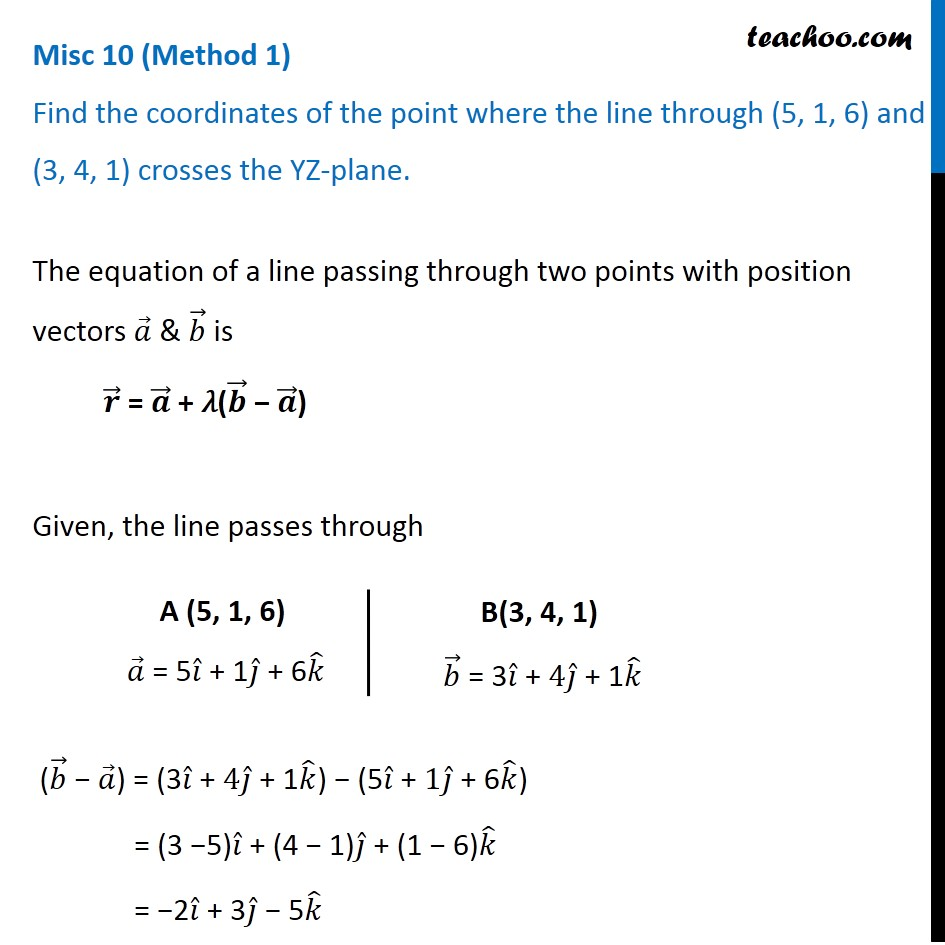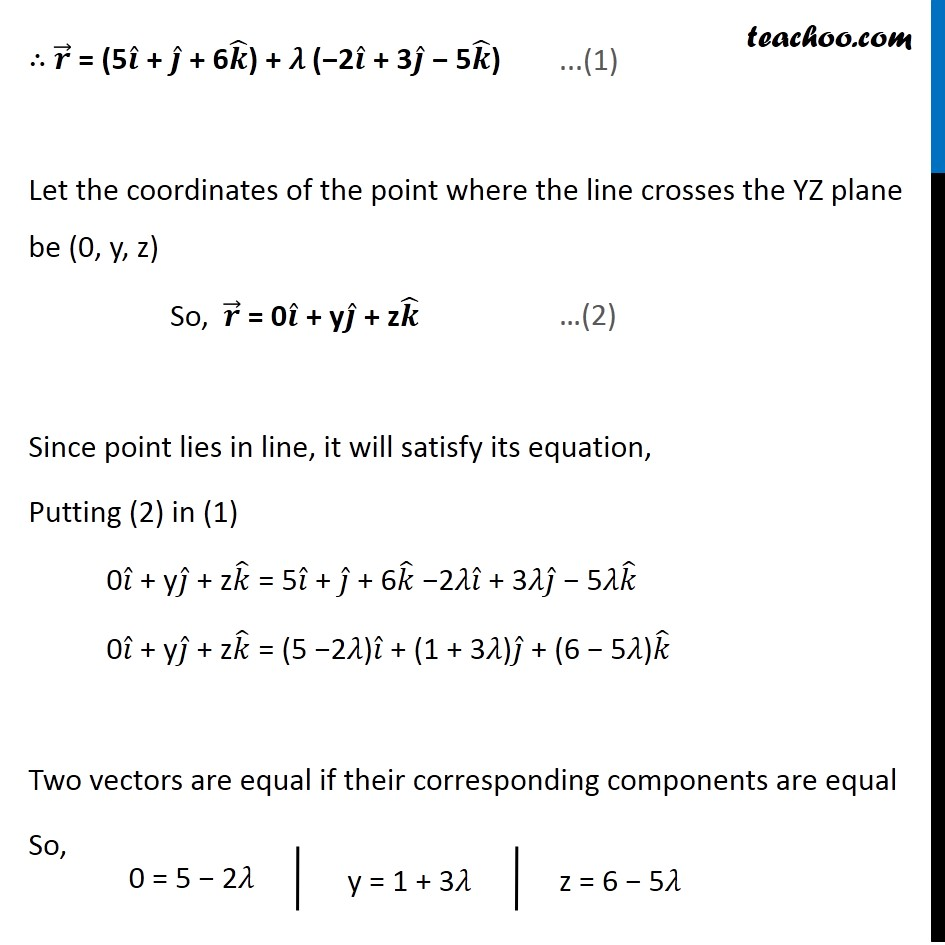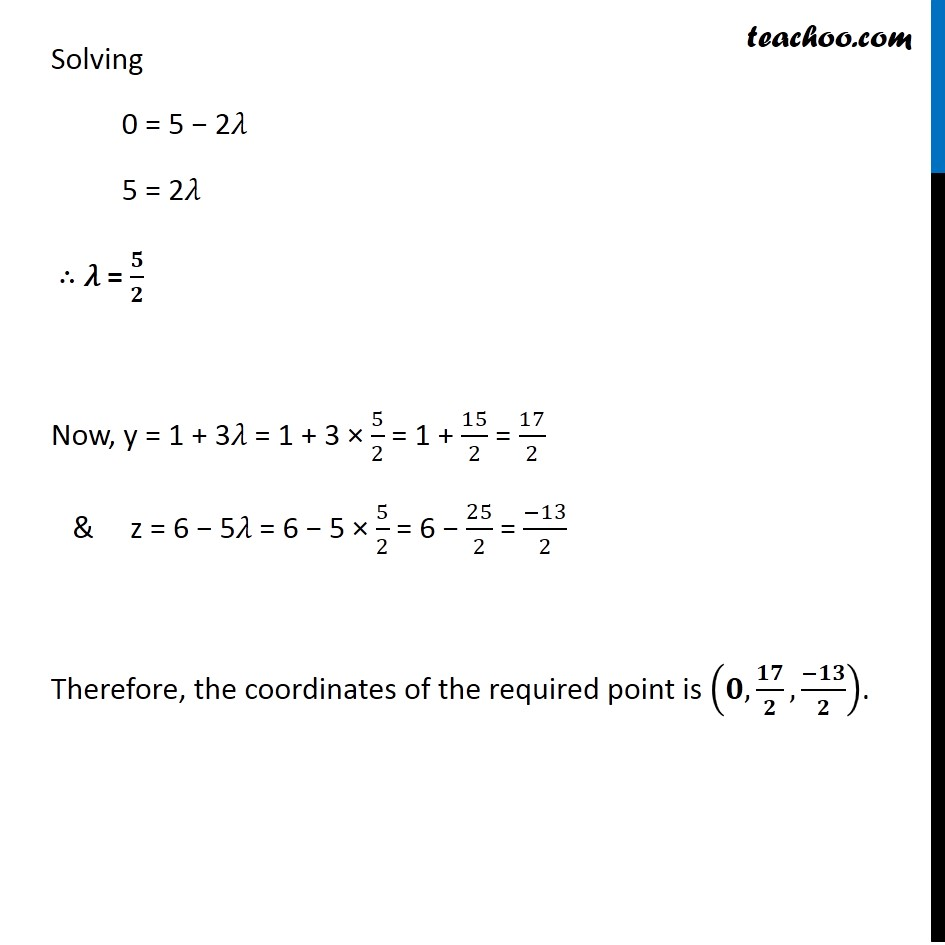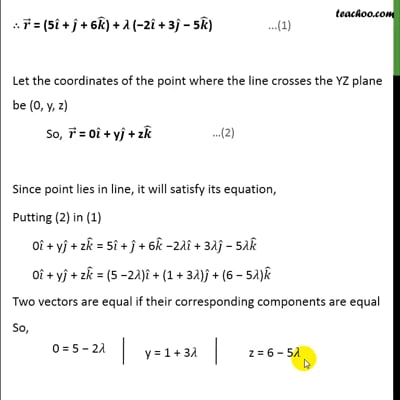This video is only available for Teachoo black users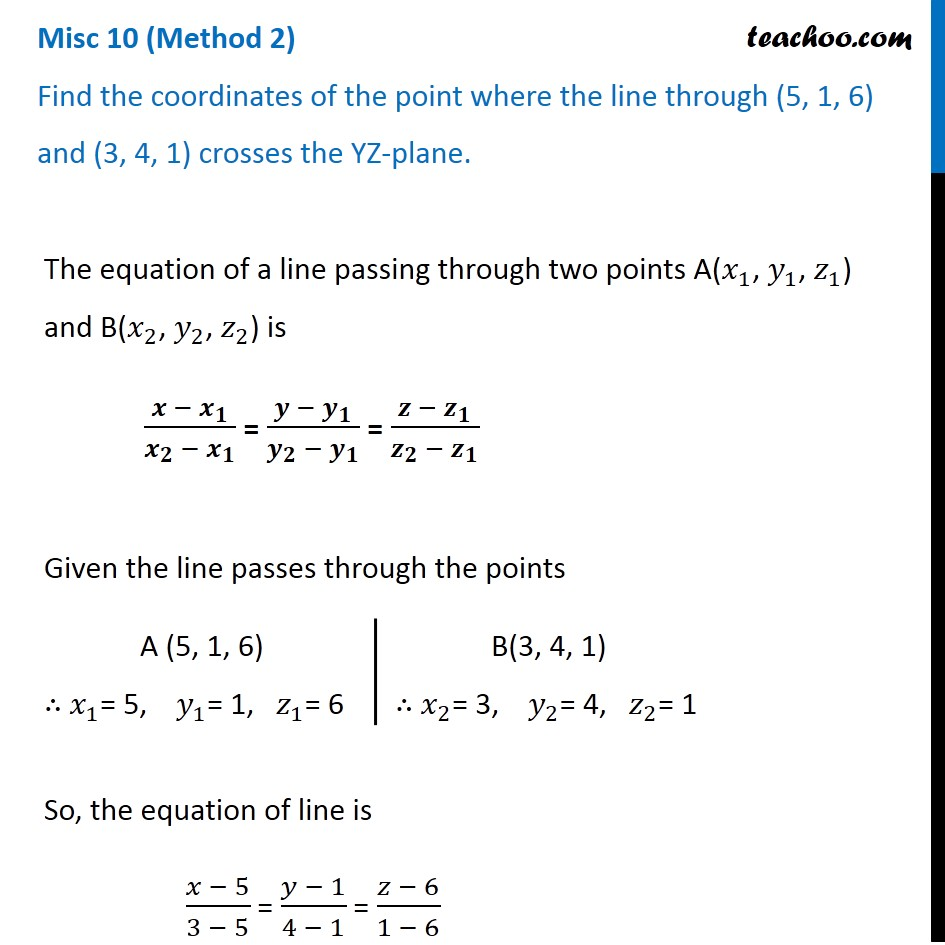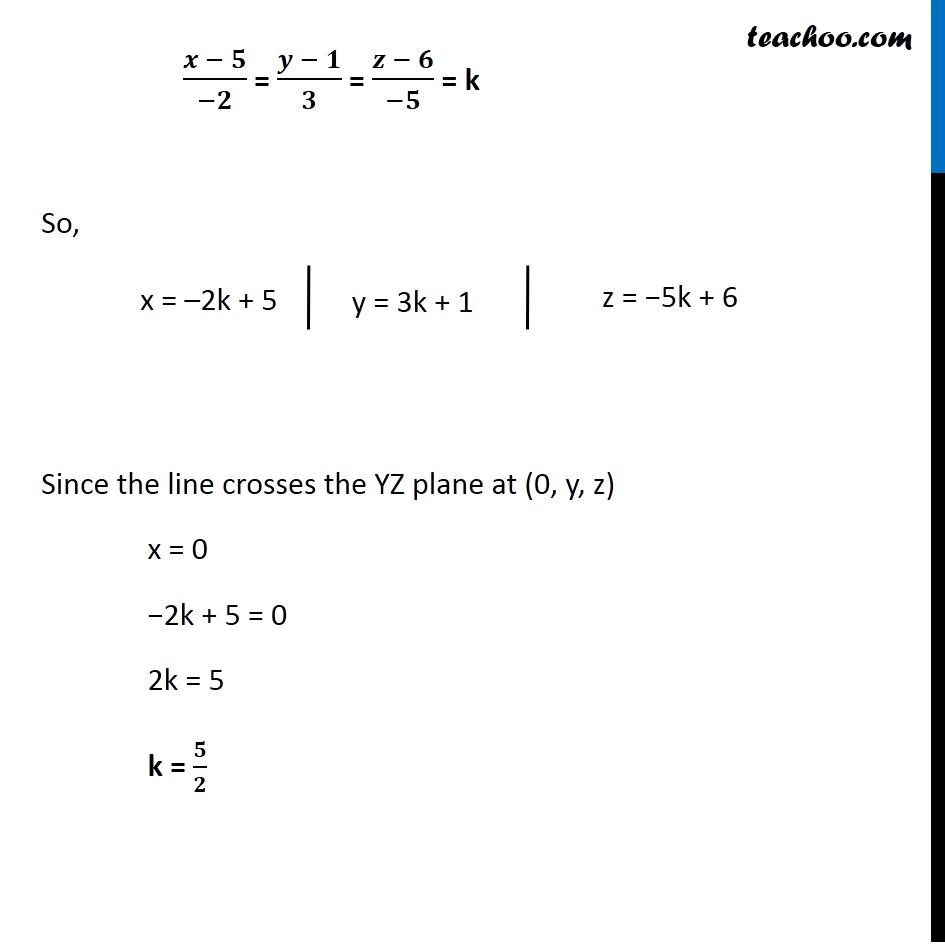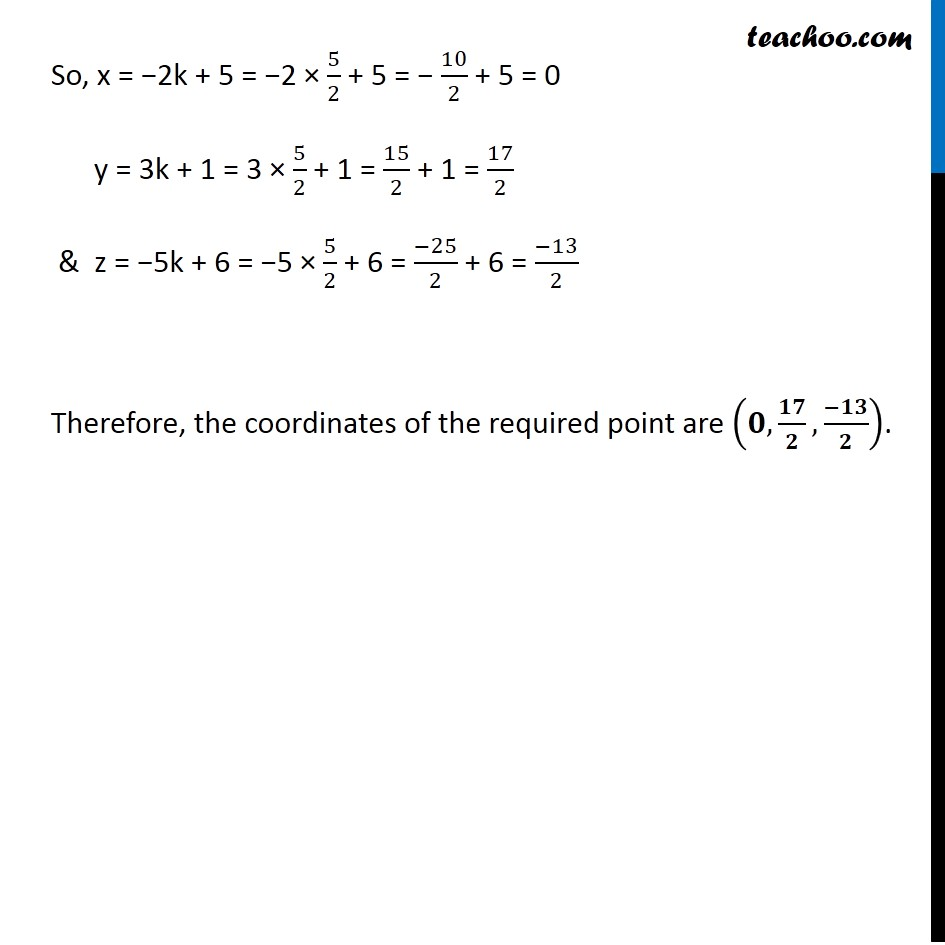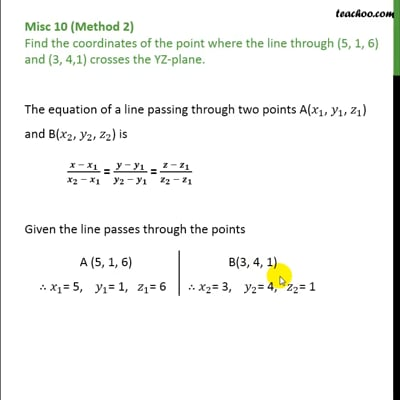This video is only available for Teachoo black users

Learn in your speed, with individual attention - Teachoo Maths 1-on-1 Class

### Transcript

Question 6 (Method 1) Find the coordinates of the point where the line through (5, 1, 6) and (3, 4, 1) crosses the YZ-plane.The equation of a line passing through two points with position vectors 𝑎 ⃗ & 𝑏 ⃗ is 𝒓 ⃗ = 𝒂 ⃗ + 𝜆(𝒃 ⃗ − 𝒂 ⃗) Given, the line passes through (𝑏 ⃗ − 𝑎 ⃗) = (3𝑖 ̂ + 4𝑗 ̂ + 1𝑘 ̂) − (5𝑖 ̂ + 1𝑗 ̂ + 6𝑘 ̂) = (3 −5)𝑖 ̂ + (4 − 1)𝑗 ̂ + (1 − 6)𝑘 ̂ = −2𝑖 ̂ + 3𝑗 ̂ − 5𝑘 ̂ The equation of a line passing through two points with position vectors 𝑎 ⃗ & 𝑏 ⃗ is 𝒓 ⃗ = 𝒂 ⃗ + 𝜆(𝒃 ⃗ − 𝒂 ⃗) Given, the line passes through (𝑏 ⃗ − 𝑎 ⃗) = (3𝑖 ̂ + 4𝑗 ̂ + 1𝑘 ̂) − (5𝑖 ̂ + 1𝑗 ̂ + 6𝑘 ̂) = (3 −5)𝑖 ̂ + (4 − 1)𝑗 ̂ + (1 − 6)𝑘 ̂ = −2𝑖 ̂ + 3𝑗 ̂ − 5𝑘 ̂ B(3, 4, 1) 𝑏 ⃗ = 3𝑖 ̂ + 4𝑗 ̂ + 1𝑘 ̂ ∴ 𝒓 ⃗ = (5𝒊 ̂ + 𝒋 ̂ + 6𝒌 ̂) + 𝜆 (−2𝒊 ̂ + 3𝒋 ̂ − 5𝒌 ̂) Let the coordinates of the point where the line crosses the YZ plane be (0, y, z) So, 𝒓 ⃗ = 0𝒊 ̂ + y𝒋 ̂ + z𝒌 ̂ Since point lies in line, it will satisfy its equation, Putting (2) in (1) 0𝑖 ̂ + y𝑗 ̂ + z𝑘 ̂ = 5𝑖 ̂ + 𝑗 ̂ + 6𝑘 ̂ −2𝜆𝑖 ̂ + 3𝜆𝑗 ̂ − 5𝜆𝑘 ̂ 0𝑖 ̂ + y𝑗 ̂ + z𝑘 ̂ = (5 −2𝜆)𝑖 ̂ + (1 + 3𝜆)𝑗 ̂ + (6 − 5𝜆)𝑘 ̂ Two vectors are equal if their corresponding components are equal So, Solving 0 = 5 − 2𝜆 5 = 2𝜆 ∴ 𝜆 = 𝟓/𝟐 Now, y = 1 + 3𝜆 = 1 + 3 × 5/2 = 1 + 15/2 = 17/2 & z = 6 − 5𝜆 = 6 − 5 × 5/2 = 6 − 25/2 = (−13)/2 Therefore, the coordinates of the required point is (𝟎,𝟏𝟕/𝟐, (−𝟏𝟑)/𝟐). Question 6 (Method 2) Find the coordinates of the point where the line through (5, 1, 6) and (3, 4, 1) crosses the YZ-plane.The equation of a line passing through two points A(𝑥_1, 𝑦_1, 𝑧_1) and B(𝑥_2, 𝑦_2, 𝑧_2) is (𝒙 − 𝒙_𝟏)/(𝒙_𝟐 − 𝒙_𝟏 ) = (𝒚 − 𝒚_𝟏)/(𝒚_𝟐 − 𝒚_𝟏 ) = (𝒛 − 𝒛_𝟏)/(𝒛_𝟐 − 𝒛_𝟏 ) Given the line passes through the points So, the equation of line is (𝑥 − 5)/(3 − 5) = (𝑦 − 1)/(4 − 1) = (𝑧 − 6)/(1 − 6) A (5, 1, 6) ∴ 𝑥_1= 5, 𝑦_1= 1, 𝑧_1= 6 B(3, 4, 1) ∴ 𝑥_2= 3, 𝑦_2= 4, 𝑧_2= 1 (𝒙 − 𝟓)/(−𝟐) = (𝒚 − 𝟏)/𝟑 = (𝒛 − 𝟔)/(−𝟓) = k So, Since the line crosses the YZ plane at (0, y, z) x = 0 −2k + 5 = 0 2k = 5 k = 𝟓/𝟐 x = –2k + 5 So, x = −2k + 5 = −2 × 5/2 + 5 = − 10/2 + 5 = 0 y = 3k + 1 = 3 × 5/2 + 1 = 15/2 + 1 = 17/2 & z = −5k + 6 = −5 × 5/2 + 6 = (−25)/2 + 6 = (−13)/2 Therefore, the coordinates of the required point are (𝟎,𝟏𝟕/𝟐,(−𝟏𝟑)/𝟐).